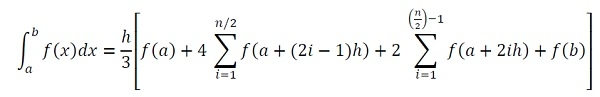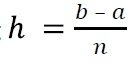# Simpson's 1/3 Rule for definite integral

Like the Trapezoidal Rule, Simpson’s 1/3rd rule is also used to find the integral value from the range a to b. The main difference between trapezoidal and the Simpson’s 1/3rd rule is, in the trapezoidal rule, the whole sections are divided into some trapezoids, but in this case, each trapezoid are also divided into two parts.

For this rule, we will follow this formula:Here h is the width of the interval, and n is the number of intervals. We can find the h by using## Input and Output

Input:
The function f(x): (x+(1/x). The lower and upper limit: 1, 2. The number of intervals: 20.
Output:
The answer is: 2.19315

## Algorithm

integrateSimpson(a, b, n)

Input − The lower and upper limit of the integral and number of intervals n.

Output − The result after integration.

Begin
h := (b - a)/n
res := f(a) + f(b)
lim := n/2

for i := 1 to lim, do
oddSum := oddSum + f(a + (2i - 1)h)
done

oddSum := oddSum * 4
for i := 1 to lim-1, do
evenSum := evenSum + f(a + 2ih)
done

evenSum := evenSum * 2
res := res + oddSum + evenSum
res := res * (h/3)
return res
End

## Example

#include<iostream>
#include<cmath>
using namespace std;

float mathFunc(float x) {
return (x+(1/x));    //function 1 + 1/x
}

float integrate(float a, float b, int n) {
float h, res = 0.0, oddSum = 0.0, evenSum = 0.0, lim;
int i;
h = (b-a)/n;    //calculate the distance between two interval
res = (mathFunc(a)+mathFunc(b));    //initial sum using f(a) and f(b)
lim = n/2;

for(i = 1; i<=lim; i++)
oddSum += mathFunc(a+(2*i-1)*h);    //sum of numbers, placed at odd number
oddSum *= 4;    //odd sum are multiplied by 4

for(i = 1; i<lim; i++)
evenSum += mathFunc(a+(2*i)*h);    //sum of numbers, placed at even number
evenSum *= 2;    //even sum are multiplied by 2
res += oddSum+evenSum;
res *= (h/3);
return res;    //The result of integration
}

main() {
float result, lowLim, upLim;
int interval;
cout << "Enter Lower Limit, Upper Limit and interval: ";
cin >>lowLim >>upLim >>interval;
result = integrate(lowLim, upLim, interval);
cout << "The answer is: " << result;
}

## Output

Enter Lower Limit, Upper Limit and interval: 1 2 20
The answer is: 2.19315